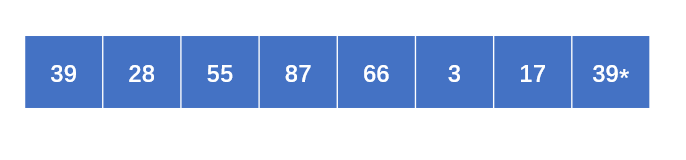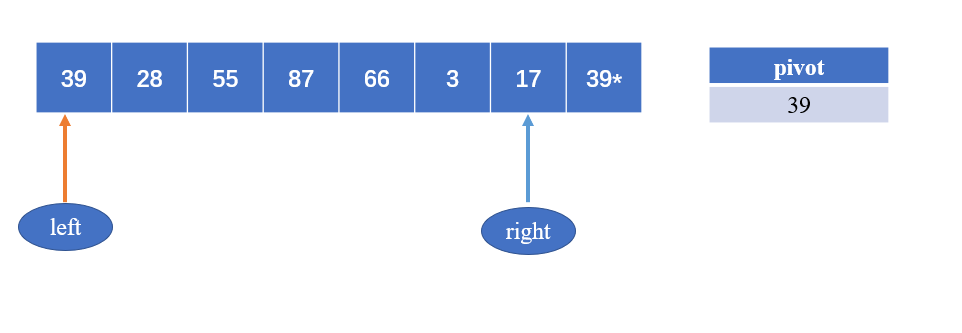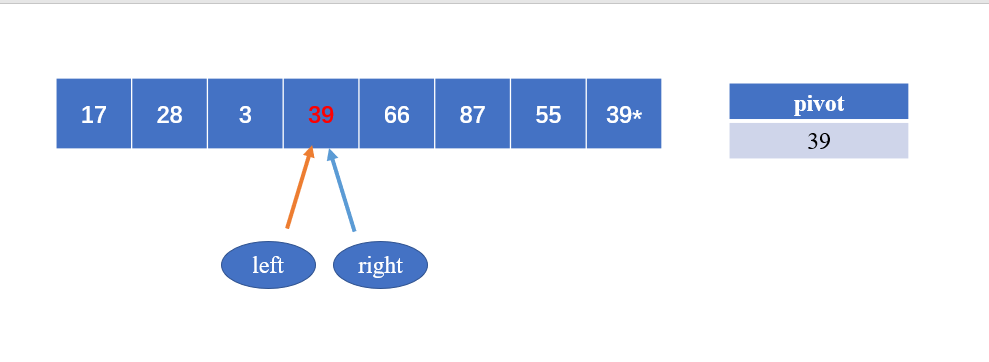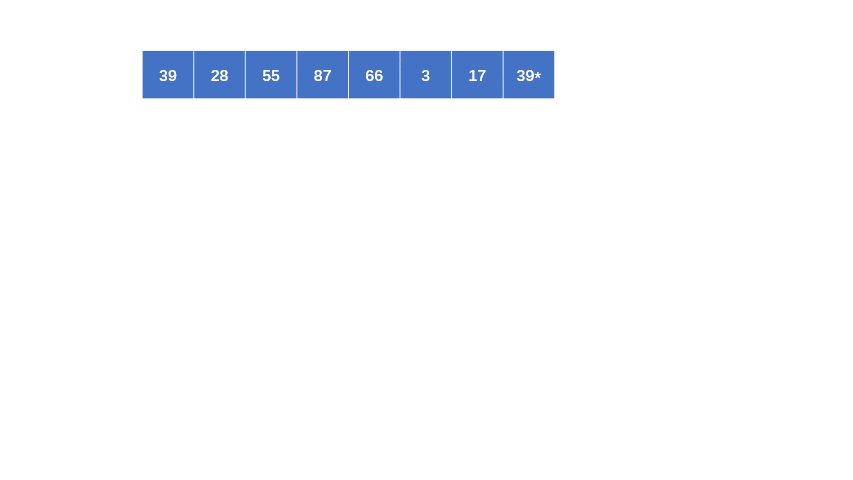# 详解快速排序

2020年4月13日15:16:45评论67阅读模式

### 例子

arr 为 [39 , 28 , 55 , 87 , 66 , 3 ,17 ,39][17,28,3] 与 [66, 87, 55, 39*]两部分；再对这两部分进行上述环节即可。

### 代码

``````public static int partition(int[] arr,int left,int right){
int pivot = arr[left];
while(left < right){
while(left<right && arr[right] >= pivot)
right--;
arr[left] = arr[right];
while(left < right && arr[left]<= pivot)
left++;
arr[right] = arr[left];
}
arr[left] = pivot;
return left;
}
``````

``````public static void quickSort(int[] arr){
quickSort(arr,0,arr.length-1);
System.out.println(Arrays.toString(arr));
}
public static void quickSort(int[] arr,int left,int right){
int middle;
if(left < right){
middle = partition(arr,left,right);
quickSort(arr,left,middle-1);
quickSort(arr,middle+1,right);
}
}
``````

``````import java.util.Arrays;

public class Solution {
public static void main(String[] args) {
quickSort(new int[]{39,28,55,87,66,3,17,39});
}

public static void quickSort(int[] arr){
quickSort(arr,0,arr.length-1);
System.out.println(Arrays.toString(arr));
}
public static void quickSort(int[] arr,int left,int right){
int middle;
if(left < right){
middle = partition(arr,left,right);
quickSort(arr,left,middle-1);
quickSort(arr,middle+1,right);
}
}

public static int partition(int[] arr,int left,int right){
int pivot = arr[left];
while(left < right){
while(left<right && arr[right] >= pivot)
right--;
arr[left] = arr[right];
while(left < right && arr[left]<= pivot)
left++;
arr[right] = arr[left];
}
arr[left] = pivot;
return left;
}
}
``````

### 稳定性

[ 2 , 3 , 1, 1* ]

[1* , 1, 2, 3]

[1* , 1 , 2 , 3]

### 欢迎关注文章源自随机的未知-https://sjdwz.com/11133.html

• 本文由 发表于 2020年4月13日15:16:45
• 转载请注明：来源：随机的未知 本文链接https://sjdwz.com/11133.html